# cross curve

## quartic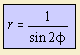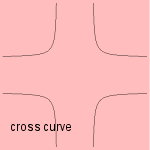The cross curve can be constructed in the following way.
Given a ellipse H, construct for every tangent the intersection with both x-axis and y-axis, giving points A and B respectively. Then draw a line l through A parallel to the y-axis, and a line m through B parallel to the x-axis. Then the intersection of line l and line m lies on the cross curve.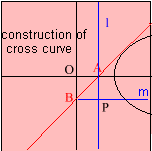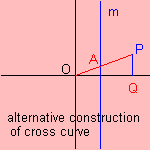This definition is a variation of the definition of the bullet nose. As is also to been seen at the curve's formula: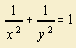r = xy is a mixed polar-Cartesian equation for the curve.

The curve can be constructed in an alternative way as follows.
Given a vertical line m. The cross curve is formed by the points P for which the distance to the x-axis is equal to the distance from the origin to the crossing of OP and m (PQ = OA in picture).

Other names for the curve are: (equilateral) cruciform (curve), stauroid and the policeman on point-duty curve.

The curve is a Lamé curve, and also an epi spiral.

The curve has been studied by Terquem (1847) and Schoute (1883).